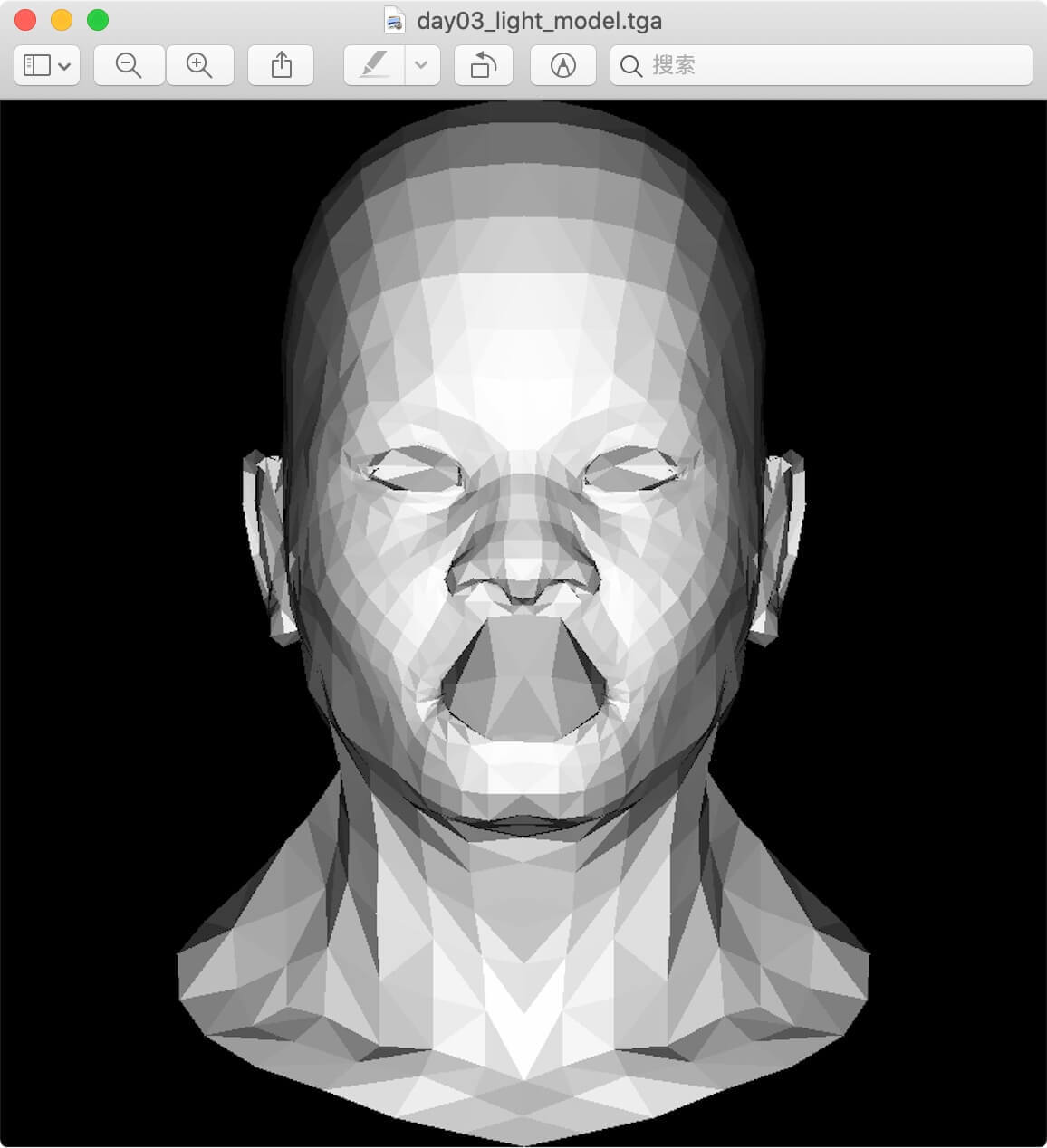# day3：画一个三角形

## 1.如何画一个三角形？#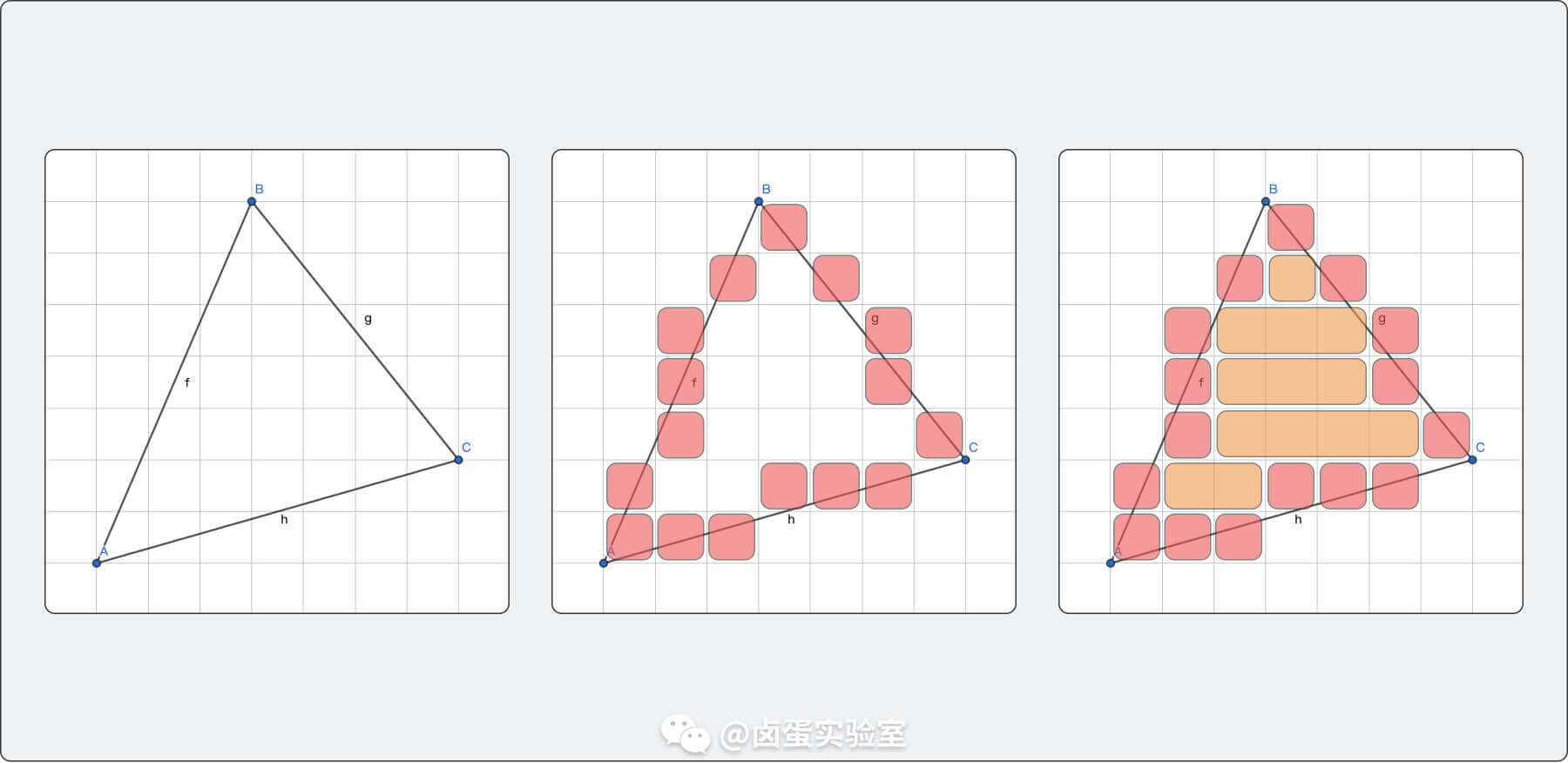## 2.利用向量叉乘画三角形#

### 2.1 数学推导#$\mathbf {a} \times \mathbf {b} =\|\mathbf {a} \|\|\mathbf {b} \|\sin(\theta )\ \mathbf {n}$

• $\mathbf {a}$ 向量叉乘 $\mathbf {b}$ 向量，如果值为，则表示 $\mathbf {b}$ 向量在 $\mathbf {a}$ 向量
• $\mathbf {a}$ 向量叉乘 $\mathbf {b}$ 向量，如果值为，则表示 $\mathbf {b}$ 向量在 $\mathbf {a}$ 向量
• $\mathbf {a}$ 向量叉乘 $\mathbf {b}$ 向量，如果值为，则表示 $\mathbf {b}$ 向量与 $\mathbf {a}$ 向量共线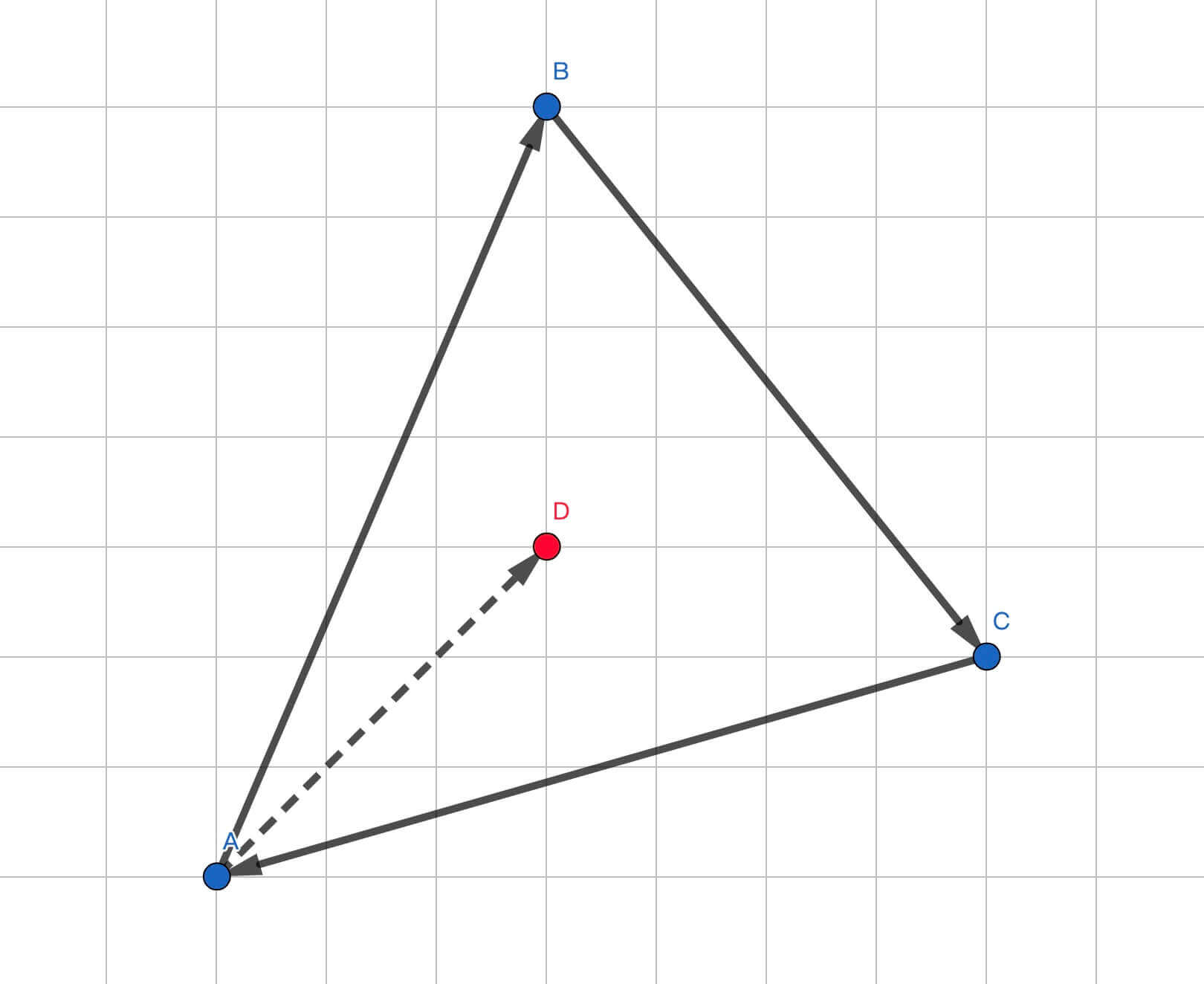• $\overrightarrow{A B} \times \overrightarrow{A P}$，值为，故 $P$$AB$ 左侧
• $\overrightarrow{B C} \times \overrightarrow{B P}$，值为，故 $P$$BC$ 左侧
• $\overrightarrow{C A} \times \overrightarrow{C P}$，值为，故 $P$$AC$ 左侧

### 2.2 代码实现#

// 利用叉乘判断是否在三角形内部
Vec3i crossProduct(Vec2i *pts, Vec2i P) {
// 构建出三角形 ABC 三条边的向量
Vec2i AB(pts.x - pts.x, pts.y - pts.y);
Vec2i BC(pts.x - pts.x, pts.y - pts.y);
Vec2i CA(pts.x - pts.x, pts.y - pts.y);
// 三角形三个顶点和 P 链接形成的向量
Vec2i AP(P.x - pts.x, P.y - pts.y);
Vec2i BP(P.x - pts.x, P.y - pts.y);
Vec2i CP(P.x - pts.x, P.y - pts.y);
return Vec3i(AB^AP, BC^BP, CA^CP);
}

void drawSingleTriangle() {
// 图片的宽高
int width = 200;
int height = 200;
TGAImage frame(width, height, TGAImage::RGB);
Vec2i pts = {Vec2i(10, 10), Vec2i(150, 30), Vec2i(70, 160)};
Vec2i P;
// 遍历图片中的所有像素
for (P.x = 0; P.x <= width - 1; P.x++) {
for (P.y = 0; P.y <= height - 1; P.y++) {
Vec3i bc_screen = crossProduct(pts, P);
// bc_screen 某个分量小于 0 则表示此点在三角形外（认为边也是三角形的一部分）
if (bc_screen.x<0 || bc_screen.y<0 || bc_screen.z<0) {
continue;
}
image.set(P.x, P.y, color);
}
}
frame.flip_vertically();
frame.write_tga_file("output/day03_cross_product_triangle.tga");
}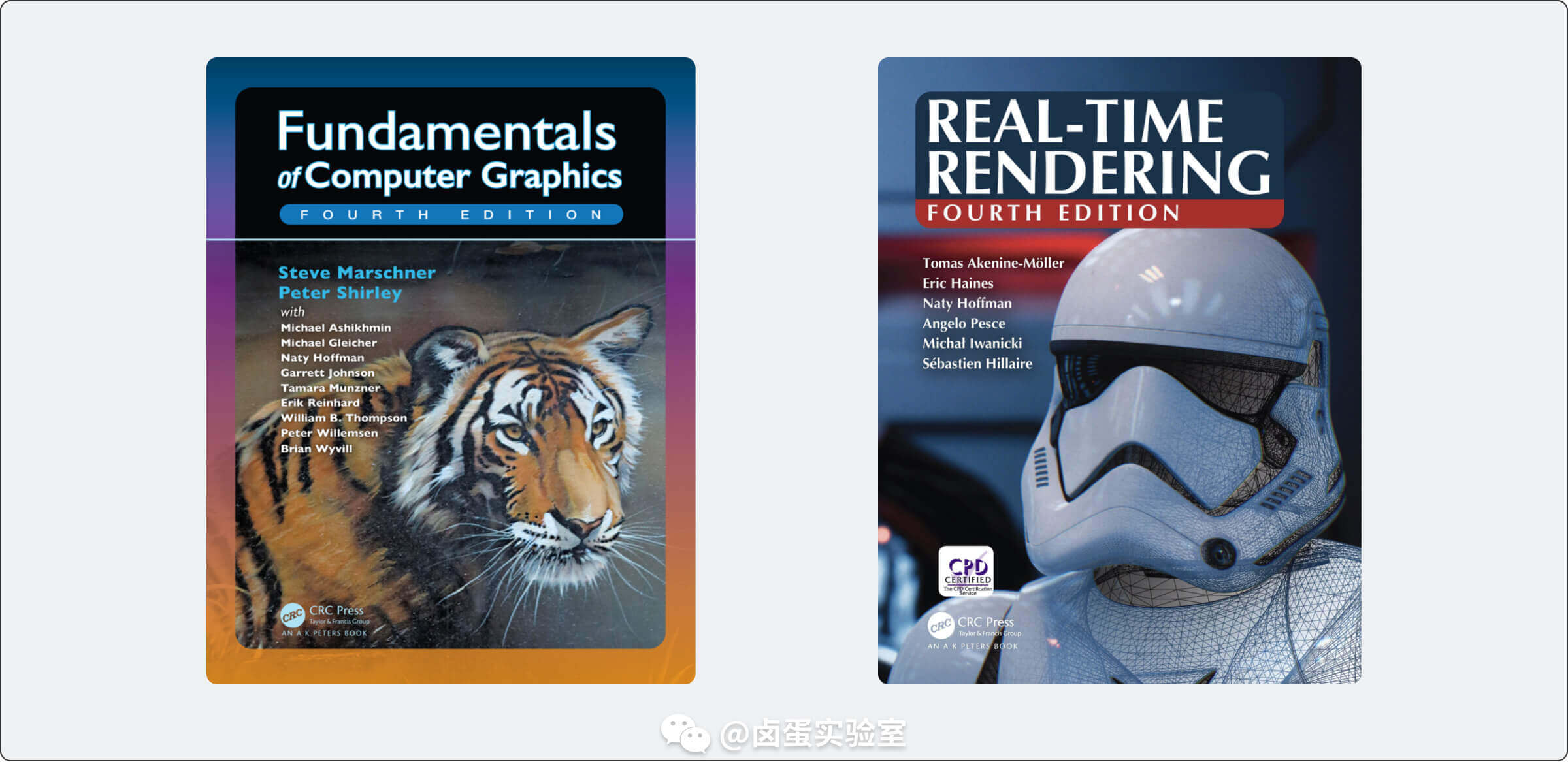## 3.利用三角形重心坐标画三角形#

### 3.1 数学推导#

$\overrightarrow{A P}=u \overrightarrow{A B}+v \overrightarrow{A C}$

$A-P=u(A-B)+v(A-C)$

$P=(1-u-v) A+u B+v C$

• $(1 - u - v)$$u$$v$大于 0 小于 1 时，P 位于三角形内部
• 一个分量等于 0 时，P 在三角形
• 两个变量等于 0 时，P 在某个顶点

$u \overrightarrow{A B}+v \overrightarrow{A C}+\overrightarrow{P A}=0$

$\left\{\begin{array}{l}u \overrightarrow{A B}_{x}+v \overrightarrow{A C}_{x}+\overrightarrow{P A}_{x}=0 \\ u \overrightarrow{A B}_{y}+v \overrightarrow{A C}_{y}+\overrightarrow{P A}_{y}=0\end{array}\right.$

$\left[\begin{array}{lll}u & v & 1\end{array}\right]\left[\begin{array}{l}\overrightarrow{A B}_{x} \\ \overrightarrow{A C}_{x} \\ \overrightarrow{P A}_{x}\end{array}\right]=0$
$\left[\begin{array}{lll}u & v & 1\end{array}\right]\left[\begin{array}{l}\overrightarrow{A B}_{y} \\ \overrightarrow{A C}_{y} \\ \overrightarrow{P A}_{y}\end{array}\right]=0$

$\mathbf{u} \times \mathbf{v}=\left|\begin{array}{cc}u_{2} & u_{3} \\ v_{2} & v_{3}\end{array}\right| \mathbf{i}-\left|\begin{array}{cc}u_{1} & u_{3} \\ v_{1} & v_{3}\end{array}\right| \mathbf{j}+\left|\begin{array}{cc}u_{1} & u_{2} \\ v_{1} & v_{2}\end{array}\right| \mathbf{k}$

$g$ 向量可以表示为这样的行列式：

$\left|\begin{array}{ll}\overrightarrow{A B}_{x} & \overrightarrow{A C}_{x} \\ \overrightarrow{A B}_{y} & \overrightarrow{A C}_{y}\end{array}\right|$

### 3.2 代码实现#

// 利用重心坐标判断点是否在三角形内部
Vec3f barycentric(Vec2i *pts, Vec2i P) {
Vec3i x(pts.x - pts.x, pts.x - pts.x, pts.x - P.x);
Vec3i y(pts.y - pts.y, pts.y - pts.y, pts.y - P.y);
// u 向量和 x y 向量的点积为 0，所以 x y 向量叉乘可以得到 u 向量
Vec3i u = x^y;
// 由于 A, B, C, P 的坐标都是 int 类型，所以 u.z 必定是 int 类型，取值范围为 ..., -2, -1, 0, 1, 2, ...
// 所以 u.z 绝对值小于 1 意味着三角形退化了，直接舍弃
if(std::abs(u.z) < 1) {
return Vec3f(-1, 1, 1);
}
return Vec3f(1.f- (u.x+u.y) / (float)u.z, u.x / (float)u.z, u.y / (float)u.z);
}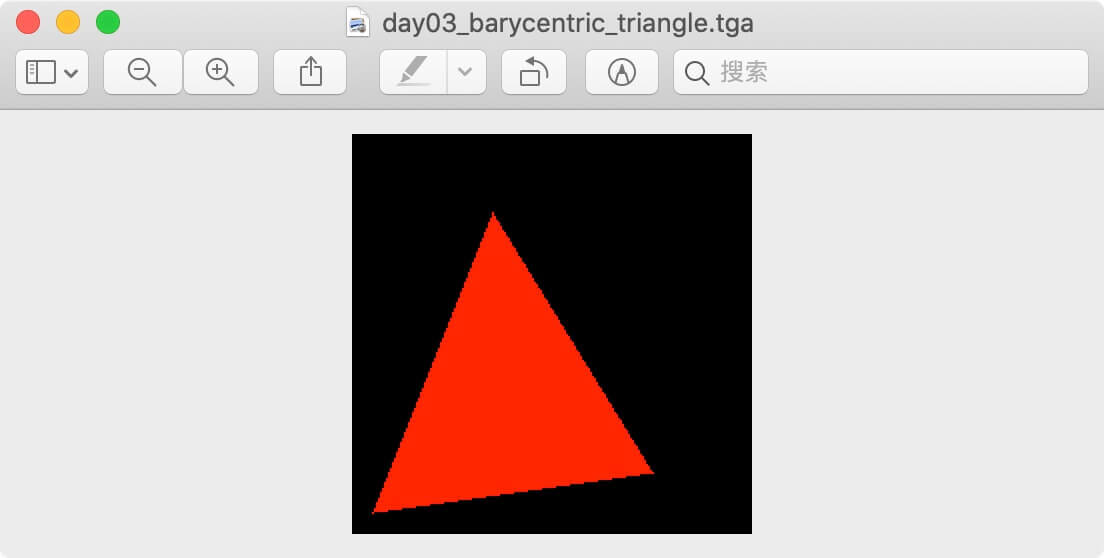## 4.绘制模型#

### 4.1 随机着色#

triangle(screen_coords, frame, TGAColor(rand() % 255, rand() % 255, rand() % 255, 255));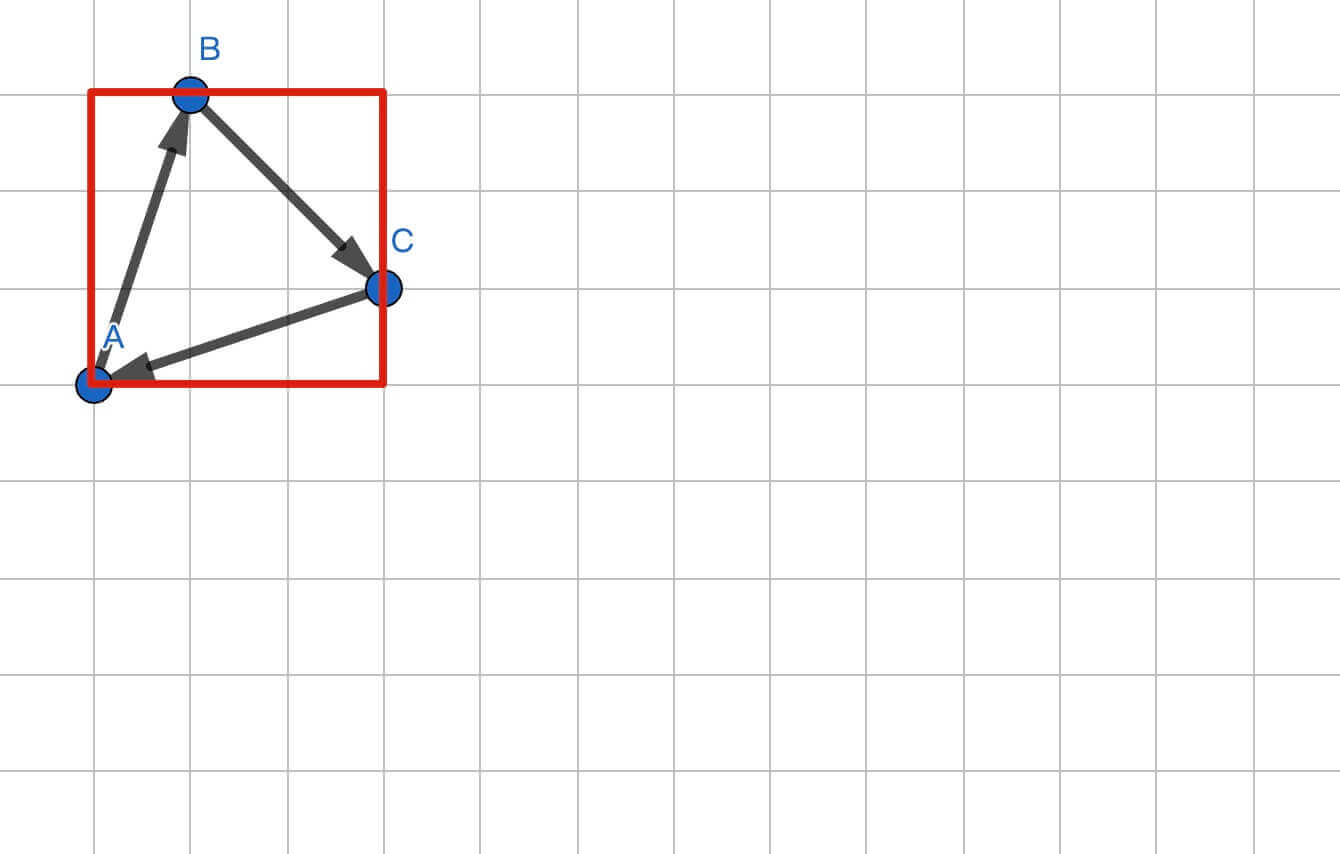// 定义包围盒
Vec2i boxmin(image.get_width() - 1, image.get_height() - 1);
Vec2i boxmax(0, 0);
// 图片的边界
Vec2i clamp(image.get_width() - 1, image.get_height() - 1);
// 查找包围盒边界
for (int i = 0; i < 3; i++) {
// 第一层循环，遍历三角形的三个顶点
for (int j = 0; j < 2; j++) {
// 第二层循环，根据顶点数值缩小包围盒的范围
boxmin.x = std::max(0, std::min(boxmin.x, pts[i].x));
boxmin.y = std::max(0, std::min(boxmin.y, pts[i].y));
boxmax.x = std::min(clamp.x, std::max(boxmax.x, pts[i].x));
boxmax.y = std::min(clamp.y, std::max(boxmax.y, pts[i].y));
}
}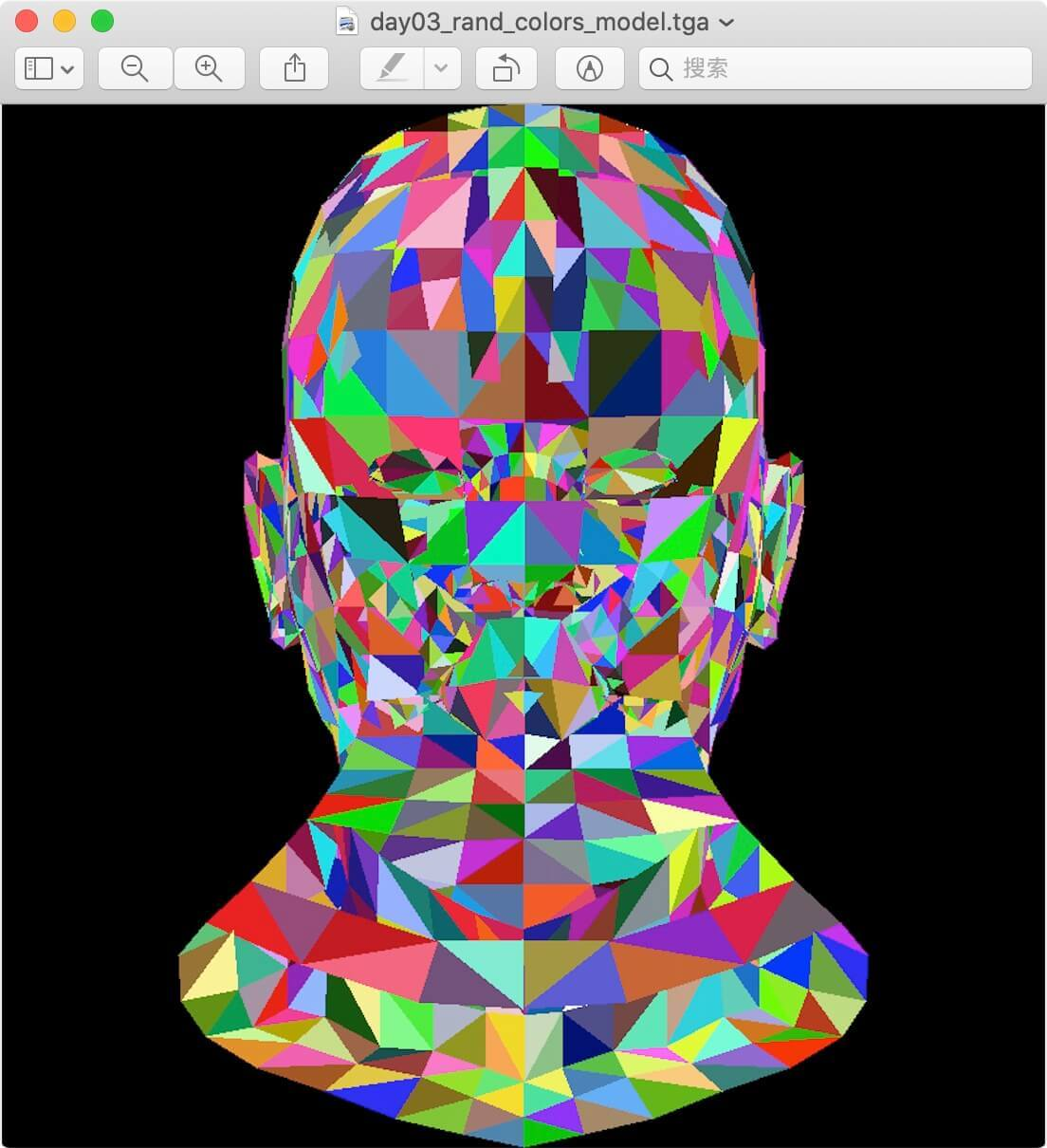### 4.2 简单的光照着色#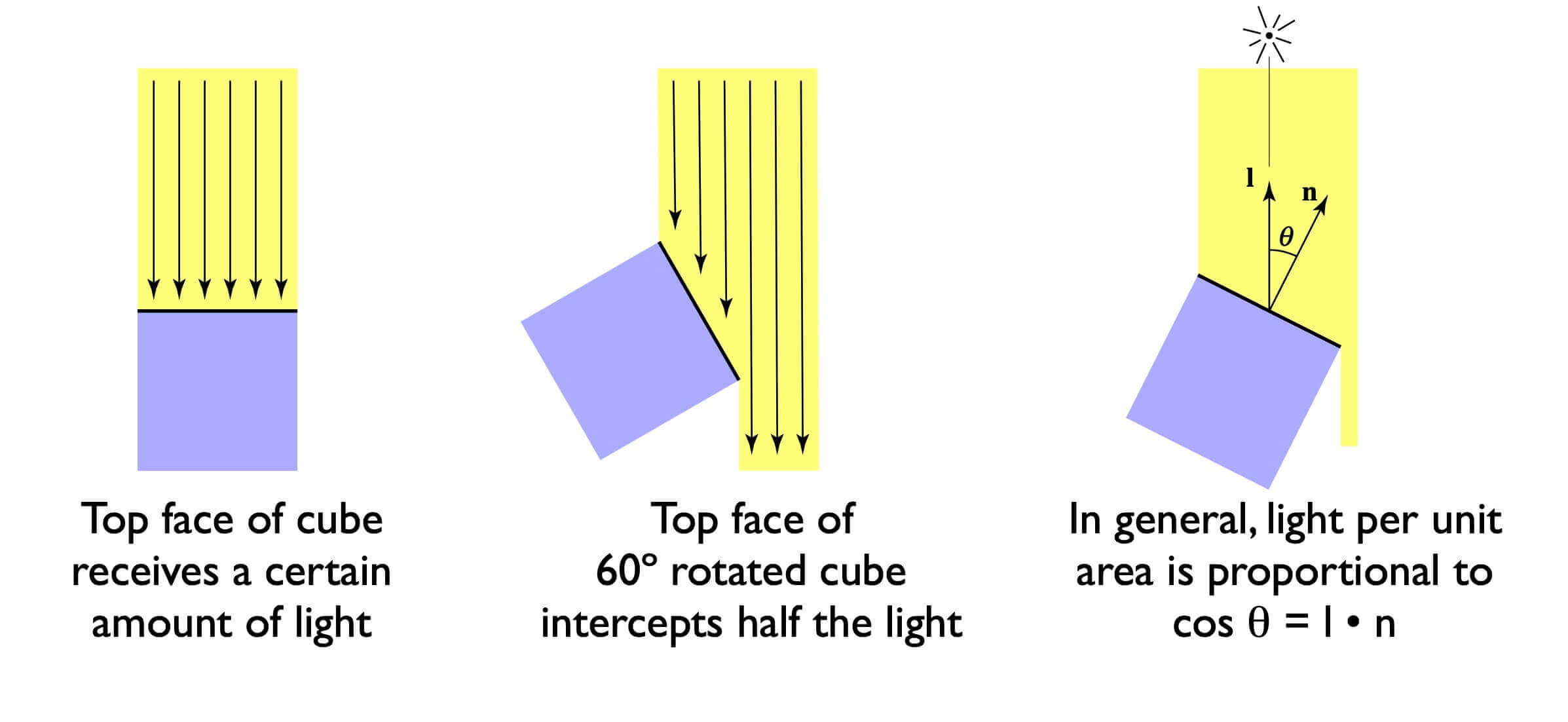• 我们要先定义一个三维空间里的光照方向（向量），然后计算三维空间里各个三角形的法线（向量）
• 两个向量归一化后，然后计算这两个向量的点乘，会得到一个值
• 这个值小于 0，说明光在三角形的另一侧，从物理上看是照射不到三角形表面的，所以直接舍弃此三角形
• 这个值大于 0，值越大，说明单位面积上接收到的光越多，三角形越亮

// 这个是用一个模拟光照对三角形进行着色
Vec3f light_dir(0, 0, -1); // 假设光是垂直屏幕的
for (int i = 0; i < model->nfaces(); i++) {
std::vector<int> face = model->face(i);
Vec2i screen_coords;
Vec3f world_coords;
// 计算世界坐标和屏幕坐标
for (int j = 0; j < 3; j++) {
Vec3f v = model->vert(face[j]);
// 投影为正交投影，而且只做了个简单的视口变换
screen_coords[j] = Vec2i((v.x + 1.) * width / 2., (v.y + 1.) * height / 2.);
world_coords[j] = v;
}
// 计算世界坐标中某个三角形的法线（法线 = 三角形任意两条边做叉乘）
Vec3f n = (world_coords - world_coords) ^ (world_coords - world_coords);
n.normalize(); // 对 n 做归一化处理
// 三角形法线和光照方向做点乘，点乘值大于 0，说明法线方向和光照方向在同一侧
// 值越大，说明越多的光照射到三角形上，颜色越白
float intensity = n * light_dir;
if (intensity > 0) {
triangle(screen_coords, frame, TGAColor(intensity * 255, intensity * 255, intensity * 255, 255));
}
}